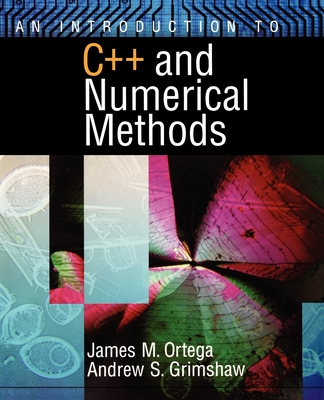An Introduction to C++ and Numerical Methods (Paperback)

Oxford University Press, USA, 9780195117677, 288pp.

Publication Date: August 6, 1998

List Price: 110.95*
* Individual store prices may vary.

Description

An Introduction to C++ and Numerical Methods provides a brief yet comprehensive introduction to programming and numerical methods for students in engineering, chemistry, physics, and applied mathematics. It is suitable for second semester or second year students who have had at least a semester of calculus.
This text offers students both an introduction to programming in C++ and clear explanations of the basics of numerical methods, including numerical integration and the solution of ordinary differential equations, nonlinear equations, and systems of linear equations. It is unique among textbooks at this level in its extensive coverage of numerical methods used in scientific and engineering computation.
An Introduction to C++ and Numerical Methods is designed to help students move quickly into writing interesting and sophisticated programs. The text begins with an introduction to scientific computing and the basic constructs of C++, including variables and assignment, typing, if statements, for and while loops, functions, one-dimensional arrays, and the cout and cin objects. After students have gained some experience with programming using these constructs, the topics are later revisited in greater detail, leading up to the important topic of classes and object-oriented programming. Throughout the text Ortega and Grimshaw emphasize the basic paradigms for constructing good programs and detecting errors.